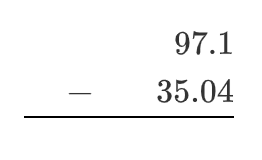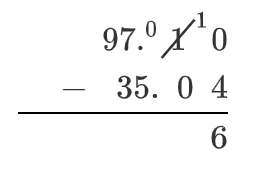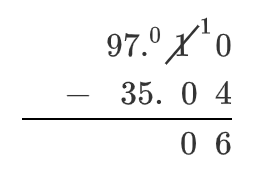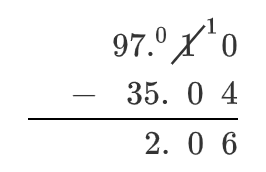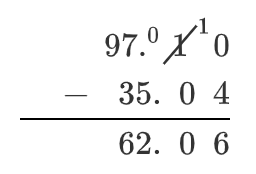### Home > CC1MN > Chapter 2 > Lesson 2.1.1 > Problem2-9

2-9.1. $9.67 + 49.7 + 5.22$

Numbers arranged in 3 rows of 5 columns as follows: Top row: blank, blank, 9, decimal, 6, 7. Middle row: 4, 9, decimal, 7, 0. Bottom row: blank, 5, decimal, 2, 2.

Add the numbers in the hundredths place. Changes: Answer row has 9 in last column.

Add the numbers in the tenths place. Since the sum is $2$ digits, place the first digit ($1$) in the column to the left. Changes: Top row: 1above the 9. Answer row: 5in fourth column.

Add the numbers in the ones place. Since the sum is $2$ digits, place the first digit ($2$) in the column to the left. Changes: Middle row has 2 above the 4. Answer row has 4 in second column and decimal in third column.

Add the numbers in the tens place. Changes: Answer row has 6 in first column.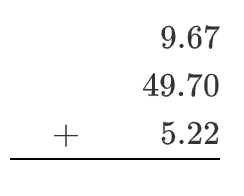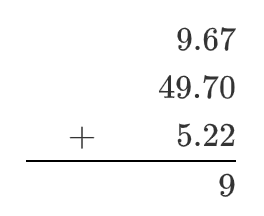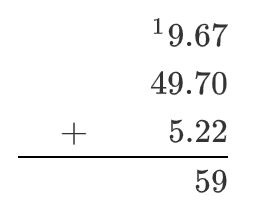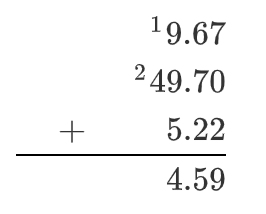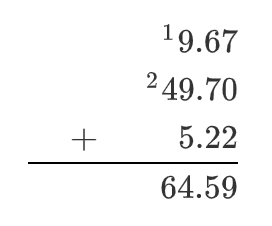1. $4.2+1.903$

See part (a). Be careful to line up the decimals correctly.

1. $97.1 - 35.04$

• Numbers arranged in 2rows of 5 columns as follows: top row: 9, 7, decimal, 1, blank. bottom row: 3, 5, decimal, 0, 4.

Since $0$ is less than $4$ in the hundredths place, borrow $1$ from the column to the left, making the hundredths place $10 − 4 = 6$Changes: top row, fourth column is 0, last column is 10. Added to answer row has, 6 in last column.

Now the tenths place is $0 − 0 = 0$Added to Answer row: 0 in fourth column.

Subtract the numbers in the ones place. Added to Answer row: 2 in second column, decimal in third column.

Subtract the numbers in the tens place. Added to answer row: 6 in first column.